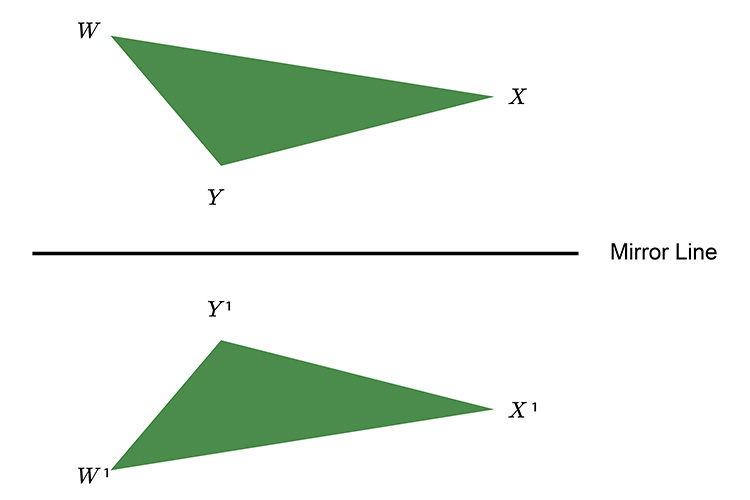# Reflection - Think mirror line

On any mathematical reflection question, think..

Where shall I put my mirror line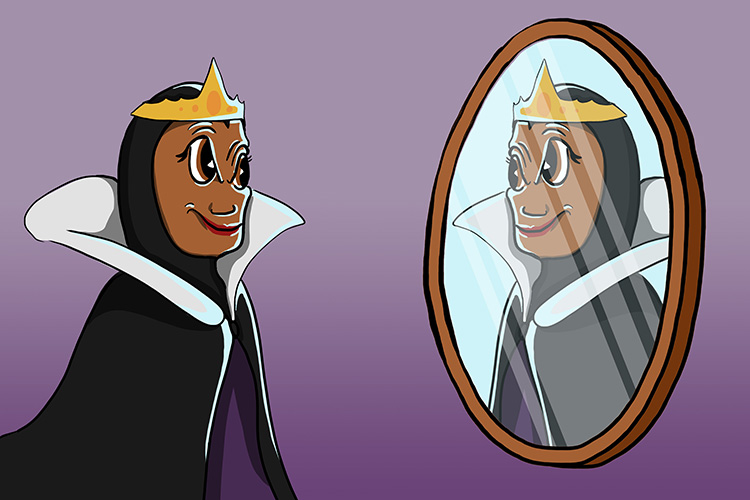Reflection think mirror.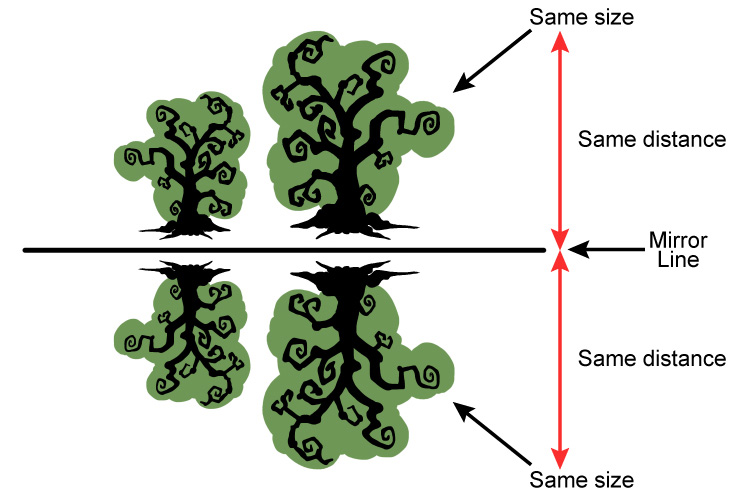On a reflection the images are the same size and the same distance from the mirror line.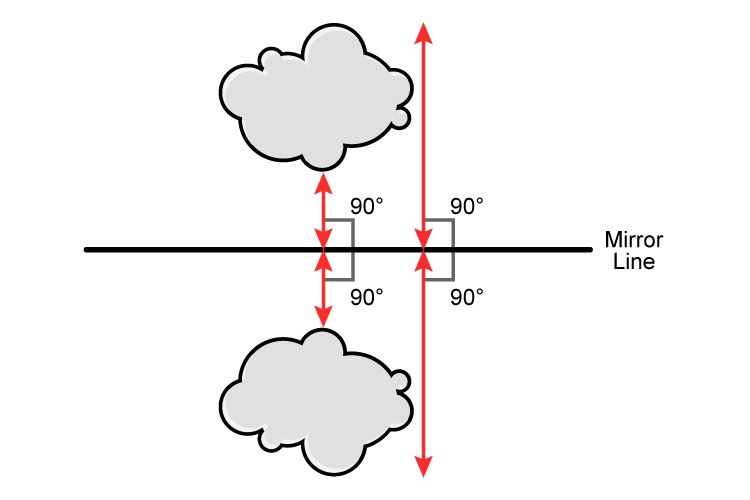SAME SIZE AND DISTANCE FROM THE MIRROR LINE.

The object and the image are perpendicular (90°) to each other.

Example

Create a reflection of the following triangle using the mirror line.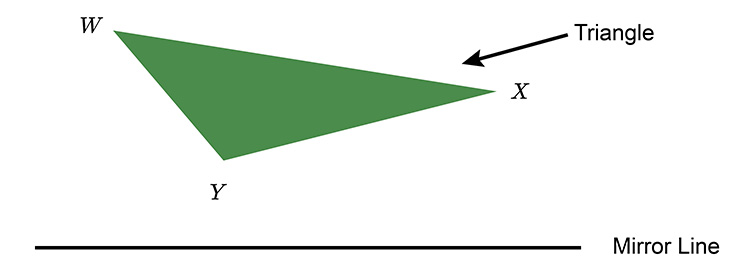We would tackle this problem by measuring each point to the mirror at 90°.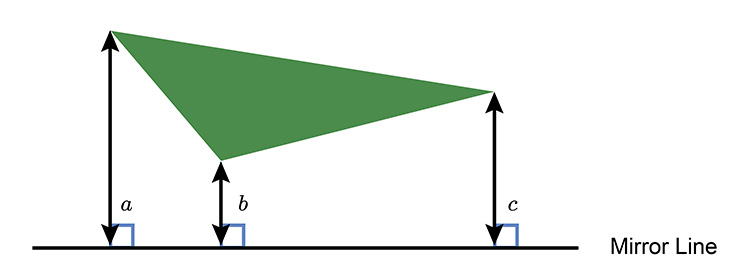Now we continue the line and measure the same distance to the other side of the mirror line.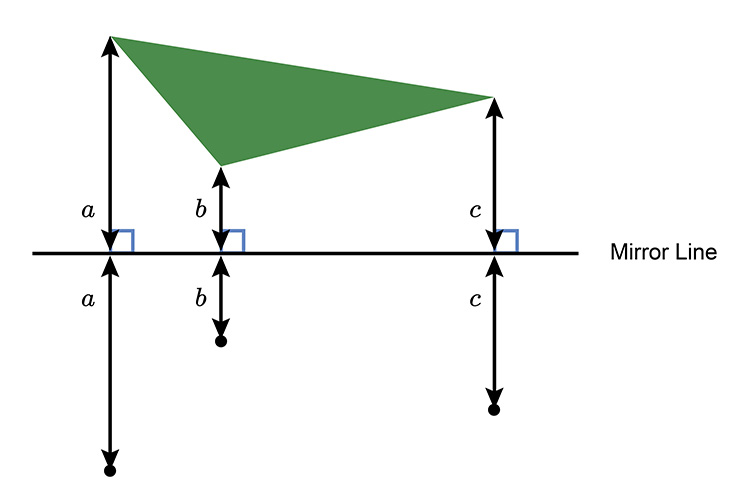Now we connect the dots.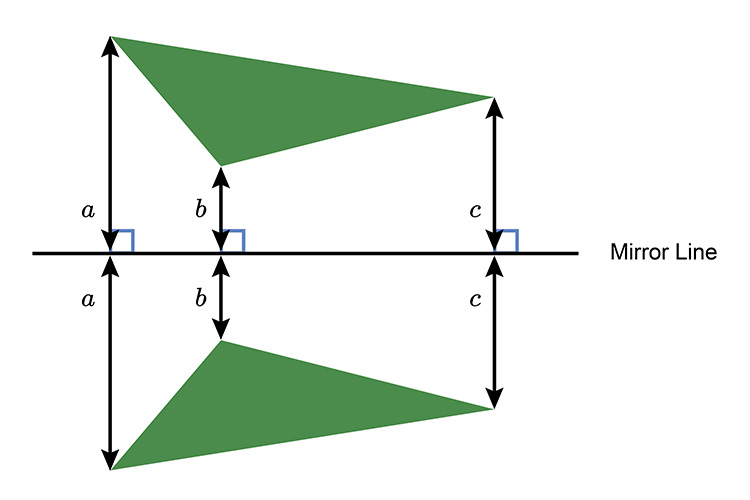This should leave you a perfect reflection: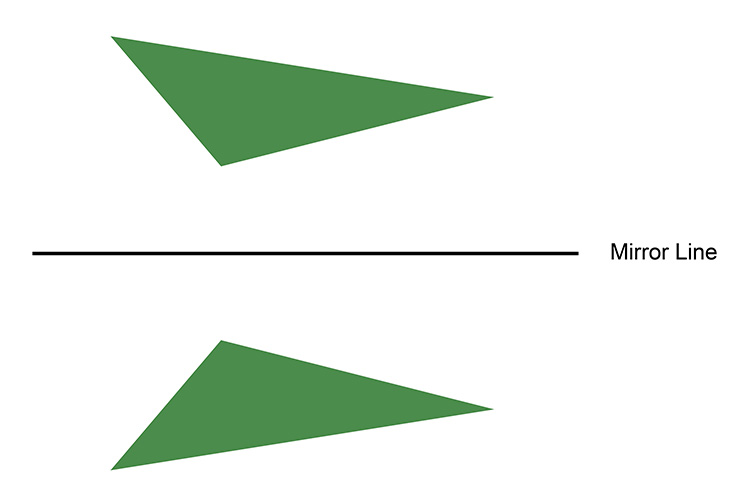We can now label the new image with the standard convention of W^1,\ X^1 \ and\ Y^1.